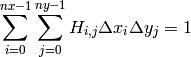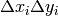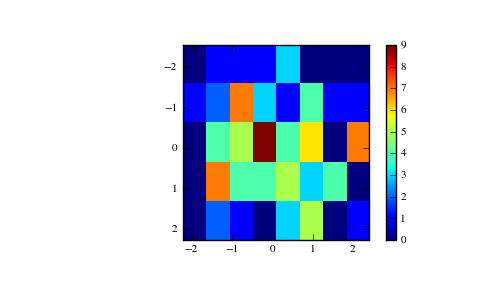# numpy.histogram2d¶

numpy.histogram2d(x, y, bins=10, range=None, normed=False, weights=None)

Compute the bi-dimensional histogram of two data samples.

Parameters : x : array_like, shape(N,) A sequence of values to be histogrammed along the first dimension. y : array_like, shape(M,) A sequence of values to be histogrammed along the second dimension. bins : int or [int, int] or array_like or [array, array], optional The bin specification: If int, the number of bins for the two dimensions (nx=ny=bins). If [int, int], the number of bins in each dimension (nx, ny = bins). If array_like, the bin edges for the two dimensions (x_edges=y_edges=bins). If [array, array], the bin edges in each dimension (x_edges, y_edges = bins). range : array_like, shape(2,2), optional The leftmost and rightmost edges of the bins along each dimension (if not specified explicitly in the bins parameters): [[xmin, xmax], [ymin, ymax]]. All values outside of this range will be considered outliers and not tallied in the histogram. normed : bool, optional If False, returns the number of samples in each bin. If True, returns the bin density, i.e. the bin count divided by the bin area. weights : array_like, shape(N,), optional An array of values w_i weighing each sample (x_i, y_i). Weights are normalized to 1 if normed is True. If normed is False, the values of the returned histogram are equal to the sum of the weights belonging to the samples falling into each bin. H : ndarray, shape(nx, ny) The bi-dimensional histogram of samples x and y. Values in x are histogrammed along the first dimension and values in y are histogrammed along the second dimension. xedges : ndarray, shape(nx,) The bin edges along the first dimension. yedges : ndarray, shape(ny,) The bin edges along the second dimension.

histogram
1D histogram
histogramdd
Multidimensional histogram

Notes

When normed is True, then the returned histogram is the sample density, defined such that:where H is the histogram array andthe area of bin {i,j}.

Please note that the histogram does not follow the Cartesian convention where x values are on the abcissa and y values on the ordinate axis. Rather, x is histogrammed along the first dimension of the array (vertical), and y along the second dimension of the array (horizontal). This ensures compatibility with histogramdd.

Examples

>>> x, y = np.random.randn(2, 100)
>>> H, xedges, yedges = np.histogram2d(x, y, bins=(5, 8))
>>> H.shape, xedges.shape, yedges.shape
((5, 8), (6,), (9,))


We can now use the Matplotlib to visualize this 2-dimensional histogram:

>>> extent = [yedges, yedges[-1], xedges[-1], xedges]
>>> import matplotlib.pyplot as plt
>>> plt.imshow(H, extent=extent, interpolation='nearest')
<matplotlib.image.AxesImage object at ...>
>>> plt.colorbar()
<matplotlib.colorbar.Colorbar instance at ...>
>>> plt.show()numpy.histogram

#### Next topic

numpy.histogramdd